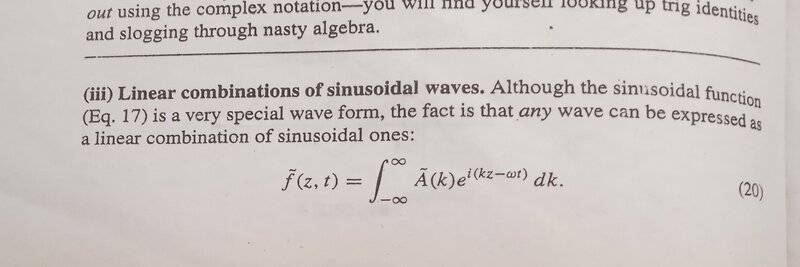# Solving a problem of chapter 9 DJ Griffith (linear combinations of sinusoidal waves)

Gold Member
Thread moved from the technical forums, and the OP has been reminded to show their work.
Homework Statement:
Obtain eq 20(show in the below picture)
Directly from the waves equation by separation of variable
Relevant Equations:
linear combinations of sinusoidal waves
While I was doing a problems of chapter 9 of DJ griffith electrodynamics

I came across this problem 4
Problem statement
Obtain eq 20(show in the below picture)
Directly from the waves equation by separation of variableCould I have a straight solution in your word
Thank you

Gold Member
2022 Award
No, but some help to find the solution yourself. Roughly speaking you just need to do two steps (which are always the same for such linear partial differential equations!):

(a) write down the wave equation
(b) write down the separation ansatz, plug it into the wave equation and find the corresponding mode functions

•vela and berkeman
Staff Emeritus